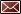Get Email Updates Back to Nonbeverage Products Laboratory page

# Drawback Tutorial

Example formulas

Simple extract

Calculating items 9 and 10 for an extract is more complex than the previous examples.Step 1. Item 9 calculation – all of the eligible alcohol comes from the 190 proof ethanol (pounds of ethanol are converted to gallons as in the previous examples): first, multiply the volume of 190 proof ethanol (8.83 gallons) by the % ethanol 8.83 gal × 0.95 = 8.39 gallons of absolute ethanol next, divide by the final yield low end of yield range 8.39 gallons of absolute ethanol ÷10.8 gallons = 0.777 or 77.7% high end of yield range 8.39 gallons of absolute ethanol ÷11.5 gallons = 0.730 or 73.0% This provides the range for the eligible alcohol in item 9. Click on the image to enlarge

Step 2. Item 10 calculation - For this, a determination of the concentration of the ethanol in the starting menstruum must be made (ethanol and water along with other dissolved components (not the oil!)).

 Ethanol = 60.0 pounds ÷ 6.7943 pounds/gallon = 8.83 gallons Water = 30.5 pounds ÷ 8.3282 pounds/gallon = 3.66 gallons Sum = ______________ 12.49 gallons

This is the volume of the starting menstruum and is used in the following equation to calculate item 10

Item 10 = (gallons of absolute ethanol) ÷ (volume (in gallons) of starting menstruum)

8.39 gallons of absolute ethanol ÷ 12.49 gallons = 0.672 or 67.2%

For extracts, the tolerance used for filtered products is applied to item 10 (see the tolerance table).

Why use the starting menstruum?

The oil does not add significant volume to the final product because it will not dissolve to an appreciable extent in the water: ethanol mixture.  Once the oil is removed, the ethanol content will be determined by the proportions of the soluble ingredients (in this case, the water and ethanol).